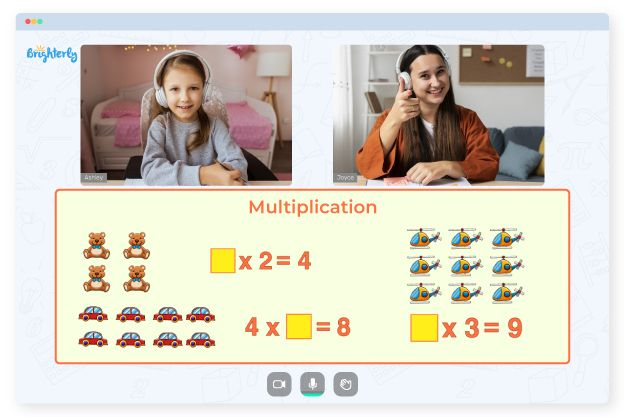# Multiplication Table Worksheets

A child’s first encounter with multiplication is the multiplication table. Using the table, kids learn everything about multiplication facts. After they have mastered the times table from 1 to 12, they move on to learn how multiplication of other numbers works.

Although the multiplication table is limited because numbers are infinite and can’t all fit into the table, the tool is an integral part of a child’s primary math education. Still, kids often need practice tests to strengthen the knowledge of math concepts, basic or not. Math worksheets help evaluate a kid’s understanding of multiplication and improve their knowledge.

## The Benefits of Multiplication Table Worksheets

With multiplication table worksheets, your child has a better chance of excelling at practice tests, no matter how complex they may seem at the beginning. But the advantages don’t stop there; times table worksheets can also help students by:

### Familiarizing them with the concept of multiplication

Multiplication can seem complex, but the use of time table worksheets encourages continuous, guided practice. With a multiplication table worksheet, students can study the problems already solved by the creators. They remember the patterns and the sequence used to derive the answers and then continue on that path using similar strategies to solve other multiplication problems.

Math for Kids

Is Your Child Struggling With Math?
1:1 Online Math Tutoring### Training them to solve math faster

If a kid wants to be a faster math solver, they don’t have to waste time on solving simple multiplication problems. Instead, they need to remember the multiplication facts by heart.

With consistent practice using times table worksheets, kids can remember answers and sequences easier. This benefit will come in handy in a timed math competition where speed is essential.

### Serving as the basis for other math topics

Fractions, decimals, division, percentages, and factorization are other math concepts that require a strong understanding of multiplication. Thus, practicing with a multiplication table worksheet is a great way to gain a strong foundation for more complex math.### Multiplication Table Worksheets PDF

Times Tables Worksheets### Multiplication Table Worksheets PDF

Multiplication Table Worksheet### Multiplication Table Worksheets PDF

Times Table Worksheets### Multiplication Table Worksheets PDF

Time Table Worksheets

## Printable Multiplication Tables Worksheets

To help your kids with multiplication tables, you need printable times table worksheets they can solve. There are many printable times table worksheets on math websites, and each is of different levels or grades. For the best results, download many times table worksheets, printable and colorful ones so that your kids have options.

An easy way to measure the metrics of your student’s growth is the use of time tables worksheets. Printable multiplication worksheets come in different types that can be used to your advantage. After downloading and printing worksheets, you can share copies with everyone in the class and see how diversity of exercises helps keep students engaged.

Additionally, you can make copies and share the same with everyone to see how students’ diverse learning styles show up when working through the worksheets. Just remember to download the colorful worksheets to keep them interested in the tasks.

### Times Tables Worksheets

Problems with Multiplication?• Is your child having trouble with understanding multiplication?
• Start learning with an online tutor.

Does your child struggle to keep up with multiplication concepts? An online tutor could provide assistance.